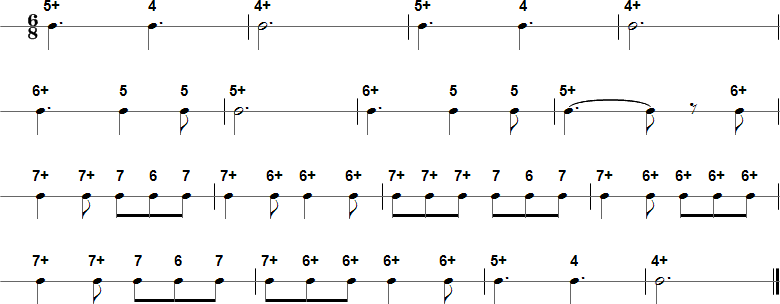Browse by Title:

# Three Blind Mice - Harmonica Tablature and Sheet Music## Tab in Text Format

5+ 4 4+ 5+ 4 4+ 6+ 5 5 5+ 6+ 5 5 5+ 6+ 7+ 7+ 7 6 7 7+ 6+ 6+ 6+ 7+ 7+ 7+ 7 6 7 7+ 6+ 6+ 6+ 6+ 7+ 7+ 7 6 7 7+ 6+ 6+ 6+ 6+ 5+ 4 4+